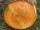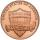# Common cylinder

I've quite common example of a rotary cylinder.
Known: S1 = 1 m2, r = 0.1 m Calculate : v =? V =?

You can verify the results?

Result

v =  1.592 m
V =  0.05 m3

#### Solution:Leave us a comment of example and its solution (i.e. if it is still somewhat unclear...):Be the first to comment!#### To solve this example are needed these knowledge from mathematics:

Tip: Our volume units converter will help you with converion of volume units.

## Next similar examples:

1. Two circlesTwo circles with a radius 4 cm and 3 cm have a center distance 0.5cm. How many common points have these circles?
2. Total displacementCalculate total displacement of the 4-cylinder engine with the diameter of the piston bore B = 6.6 cm and stroke S=2.4 cm of the piston. Help: the crankshaft makes one revolution while the piston moves from the top of the cylinder to the bottom and back.
3. The volumeThe volume of a solid cylinder is 260 cm3 the cylinder is melt down into a cuboid, whose base is a square of 5cm, calculate the height of the cuboid and the surface area of the cuboid
4. A pipeA radius of a cylindrical pipe is 2 ft. If the pipe is 17 ft long, what is its volume?
5. The potDiameter of the pot 38 cm. The height is 30 cm. How many liters of water can fit in the pot?
6. SawdustHow many cubic centimeters of wood sawdust is created by cut the tree trunk with a diameter of 66 cm and when the gap width is 5 mm?
7. Giant coinFrom coinage metal was produced giant coin and was applied so much metal, such as production of 10 million actual coins. What has this giant coin diameter and thickness, if the ratio of diameter to thickness is the same as a real coin, which has a diameter
8. Garden pondConcrete garden pond has bottom shape of a semicircle with a diameter 1.7 m and is 79 cm deep. Daddy wants make it surface. How many liters of water is in pond if watel level is 28 cm?
9. ConvaHow many liters of water fit into the shape of a cylinder with a bottom diameter 20 cm and a height 45 cm?
10. Circular poolThe 3.6-meter pool has a depth of 90 cm. How many liters of water is in the pool?
11. Collect rain waterThe garden water tank has a cylindrical shape with a diameter of 80 cm and a height of 12 dm. How many liters of water will fit into the tank?
12. Tank and waterCylindrical tank were poured with 3.5 liters of water. If tank base diameter is 3 dm, how height is water level in?
13. GreenhouseGarden plastic greenhouse is shaped half cylinder with a diameter of 6 m and base length 20 m. At least how many m2 of plastic is need to its cover?
14. The potThe pot is a cylinder with a volume of V = 7l and an inner diameter of d = 20cm. Find its depth.
15. Circle r,DCalculate the diameter and radius of the circle if it has length 52.45 cm.
16. MineWheel in traction tower has a diameter 5 m. How many meters will perform an elevator cabin if wheel rotates in the same direction 49 times?
17. FlowerbedIn the park there is a large circular flowerbed with a diameter of 12 m. Jakub circulated him ten times and the smaller Vojtoseven times. How many meters each went by and how many meters did Jakub run more than Vojta?